## Saturday, 9 August 2014

### CHAPTER 11 - MISCELLANEOUS EXAMPLES

 Example: 13
Find the sum of the series$1 + \dfrac{2}{6} + \dfrac{{2.5}}{{6.12}} + \dfrac{{2 \cdot 5 \cdot 8}}{{6 \cdot 12 \cdot 18}} +\ldots \infty$
if you are told that this corresponds to an expansion of a binomial, of the form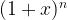${(1 + x)^n}$.
 Solution: 13
We need to determine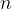$n$ and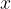$x$. For that, we can compare the terms of this series with the corresponding terms in the following general expansion.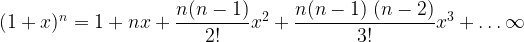${(1 + x)^n} = 1 + nx + \dfrac{{n(n - 1)}}{{2!}}{x^2} + \dfrac{{n(n - 1)\;(n - 2)}}{{3!}}{x^3} + \ldots \infty$
Thus,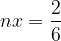$nx = \dfrac{2}{6}$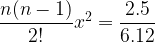$\dfrac{{n(n - 1)}}{{2!}}{x^2} = \dfrac{{2.5}}{{6.12}}$
Solving for$n$ and$x$ from these two equations, we get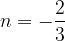$n = - \dfrac{2}{3}$ and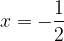$x = - \dfrac{1}{2}$. Thus, the sum of the series is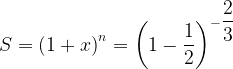$S = {\left( {1 + x} \right)^n} = {\left( {1 - \dfrac{1}{2}} \right)^{ - \dfrac{2}{3}}}$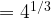$= {4^{1/3}}$
 Example: 14
Find the sum of the series$\dfrac{1}{{a + b}} + \dfrac{1}{{a + 2b}} + \dfrac{1}{{a + 3b}} + \ldots$ to$n$ terms
for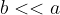$b << a$
 Solution: 14
Before solving this problem, ponder a moment over the following fact:
In the expansion of${(1 + x)^n}$, if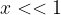$x << 1$, that is, if$x$ is much smaller than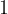$1$, then the expansion can be approximated as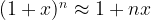${(1 + x)^n} \approx 1 + nx$
since all higher order terms can be neglected due to the small magnitude of$x$.
Coming to the problem, note that if$b << a$, i.e, if$\dfrac{b}{a} < < 1$, then,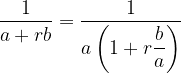$\dfrac{1}{{a + rb}} = \dfrac{1}{{a\left( {1 + r\dfrac{b}{a}} \right)}}$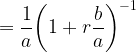$= \dfrac{1}{a}{\left( {1 + r\dfrac{b}{a}} \right)^{ - 1}}$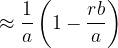$\approx \dfrac{1}{a}\left( {1 - \dfrac{{rb}}{a}} \right)$ since$\dfrac{b}{a} < < 1$
Thus, the sum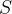$S$ of the series is (to a good approximation)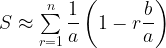$S \approx \sum\limits_{r = 1}^n {} \dfrac{1}{a}\left( {1 - r\dfrac{b}{a}} \right)$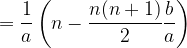$= \dfrac{1}{a}\left( {n - \dfrac{{n(n + 1)}}{2}\dfrac{b}{a}} \right)$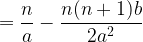$= \dfrac{n}{a} - \dfrac{{n(n + 1)b}}{{2{a^2}}}$
 Example: 15
 Evaluate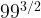${99^{3/2}}$ correct to four decimal places.
 Solution: 15
We have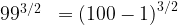${99^{3/2}}\;\; = {\left( {100 - 1} \right)^{3/2}}$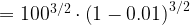$= {100^{3/2}} \cdot {\left( {1 - 0.01} \right)^{3/2}}$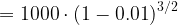$= 1000 \cdot {\left( {1 - 0.01} \right)^{3/2}}$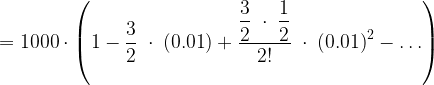$= 1000 \cdot \left( {1 - \dfrac{3}{2}\; \cdot \;\left( {0.01} \right) + \dfrac{{\dfrac{3}{2}\; \cdot \;\dfrac{1}{2}}}{{2!}}\; \cdot \;{{\left( {0.01} \right)}^2} - \ldots } \right)$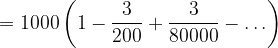$= 1000\left( {1 - \dfrac{3}{{200}} + \dfrac{3}{{80000}} - \ldots } \right)$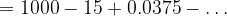$= 1000 - 15 + 0.0375 - \ldots$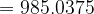$= 985.0375$
Note that we only considered the first three terms of the expansion because the higher order terms would not have had any effect on the answer up to the fourth decimal place.